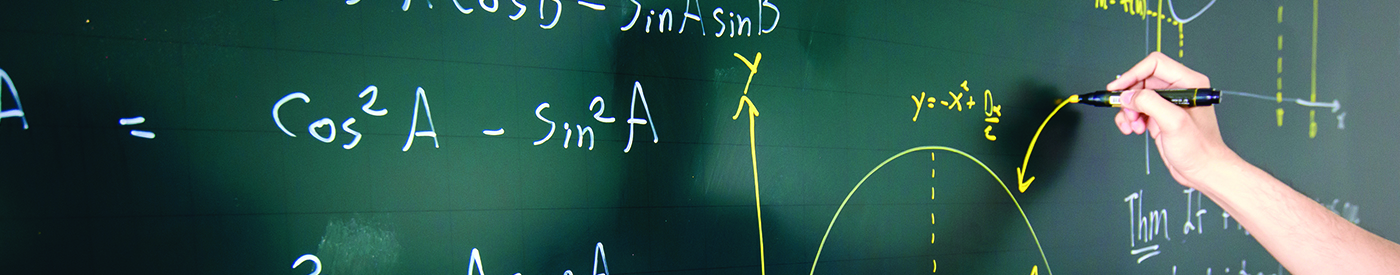## Fusion Math S1 (13 yr old)

##### Objectives
1. Generate interest in Mathematics and equip students with Mathematical knowledge.
2. Enhance students’ problem solving skills and strengthen their abilities to attempt challenging Mathematical problems similar to SMO (Junior).
3. Head start for all Primary 6 students (after their PSLE) to learn Secondary IP Math syllabus (Module 1 & 2) in advance through acquiring algebra and analytical geometry knowledge.
4. Primary 4 and 5 students (selective) who are keen to learn in advance can participate.

Semester 1 (Jan – Mar) Semester 1 (Mar – May)
– Revision on Algebraic Topics
– Word Problems (%, Rate & Speed)
– Logic Problems
– Completing the Squares
– Sum & Product of Roots
– Divisibility
– Number Theory
– Congruence
– Indices & Surds
– Algebraic Identities
– Inequalities (AM-GM)
– Geometry (Angles)
– Geometry (Lengths)
– Geometry (Areas)
– Permutation & Combinations
– Combinatorics (Counting)
– Revision Papers
Semester 2 (Jun – Sep) Semester 2 (Sep – Nov)
– AMC Practice Papers
– Number Patterns
– Series (Summation)
– Pythagoras’ Theorem
– Triangles
– Circles Properties
– Sets
– Binomial Theorem
– Algebraic Identities
– Linear Inequalities
– Discriminant & Nature of Roots
– Number Theory (Factorization)
Module 1 Semester 2 (Oct – Nov) / Year End Holiday Module 2 Semester 2 (Nov) / Year End Holiday
– Real Numbers
– Approximation & Estimation, HCF & LCM
– Basic Algebra
– Algebra Expansion
– Algebra Factorization
– Linear Equation
– Linear Graph
– Pythagoras’ Theorem
– Probability